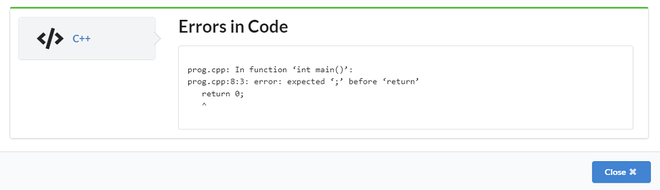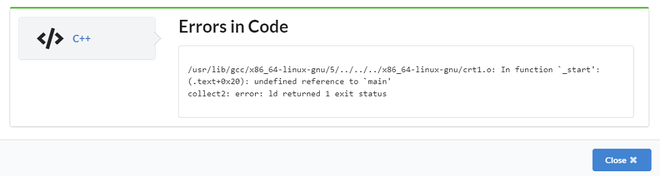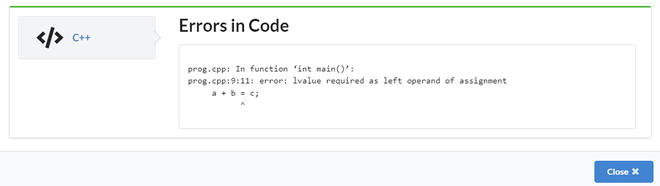GFG App
Open AppBrowser
Continue

# C++ Program to Show Types of Errors

In any programming language errors is common. If we miss any syntax like parenthesis or semicolon then we get syntax errors. Apart from this we also get run time errors during the execution of code. In a similar way the errors are classified as below:

1. Syntax Errors
2. Runtime Errors
3. Logical Errors
5. Semantic Errors

## 1. Syntax Errors

These are also referred to as compile-time errors. These errors have occurred when the rule of C++ writing techniques or syntax has been broken. These types of errors are typically flagged by the compiler prior to compilation.

Example: In the below program we are getting an error because of a missing semicolon at the end of the output statement (cout) called syntax error.

## C++

 `// C++ program to demonstrate ` `// a syntax error ` `#include ` `using` `namespace` `std; ` ` `  `int` `main() {  ` `  ``cout << ``"Geeks for geeks!"` `// missing semicolon ` `  ``return` `0;  ` `}`

Output:Syntax Error

## 2. Runtime Errors

This type of error occurs while the program is running. Because this is not a compilation error, the compilation will be completed successfully.
These errors occur due to segmentation fault when a number is divided by division operator or modulo division operator.

Example: Let us consider an array of length 5 i.e. array, but during runtime, if we try to access 10 elements i.e array then we get segmentation fault errors called runtime errors. Giving only an array length of 5

## C++

 `// C++ program to demonstrate ` `// a runtime error ` `#include ` `using` `namespace` `std; ` ` `  `int` `main() ` `{ ` ` `  `    ``int` `array; ` `    ``return` `0; ` `}`

But in output trying to access more than 5 i.e if we try to access array during runtime then we get an error.

Output:

`Segmentation fault `

## 3. Logical Errors

Even if the syntax and other factors are correct, we may not get the desired results due to logical issues. These are referred to as logical errors. We sometimes put a semicolon after a loop, which is syntactically correct but results in one blank loop. In that case, it will display the desired output.

Example: In the below example, the for loop iterates 5 times but the output will be displayed only one time due to the semicolon at the end of for loop. These kinds of errors are called logical errors.

## C++

 `// C++ program to demonstrate ` `// a logical error ` `#include ` `using` `namespace` `std; ` ` `  `int` `main() { ` `  ``int` `j; ` `  ``// Cause of Logical error ` `  ``for``(j=0;j<=5;j++); ` `  ``{ ` `    ``cout << ``"Geeks for geeks"``; ` `  ``} ` `    ``return` `0; ` `}`

Output

`Geeks for geeks`

When the program is successfully compiled and attempting to link the different object files with the main object file, errors will occur. When this error occurs, the executable is not generated. This could be due to incorrect function prototyping, an incorrect header file, or other factors. If main() is written as Main(), a linked error will be generated.

Example:

## C++

 `// C++ program to demonstrate ` `// a linker error ` `#include ` `using` `namespace` `std; ` ` `  `int` `Main() { ` ` `  `    ``cout << ``"Geeks for geeks"``; ` `    ``return` `0; ` `} `

Output:## 5. Semantic Errors

When a sentence is syntactically correct but has no meaning, semantic errors occur. This is similar to grammatical errors. If an expression is entered on the left side of the assignment operator, a semantic error may occur.

Example:

## C++

 `// C++ program to demonstrate ` `// a semantic error ` `#include ` `using` `namespace` `std; ` ` `  `int` `main() ` `{ ` `    ``int` `a = 10, b = 20, c; ` `    ``a + b = c; ` ` `  `    ``cout << c; ` `    ``return` `0; ` `}`

Output:Semantic Error

My Personal Notes arrow_drop_up